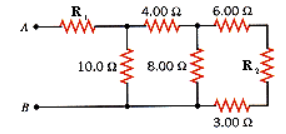# Problem: Find the equivalent resistance between points A and B in the drawing (R1 = 4.00, R2 = 8.00).

###### FREE Expert Solution

The equivalent resistance of series resistors,

$\overline{)\begin{array}{rcl}{\mathbf{R}}_{\mathbf{eq}}& {\mathbf{=}}& {\mathbf{R}}_{\mathbf{1}}\mathbf{+}{\mathbf{R}}_{\mathbf{2}}\mathbf{+}{\mathbf{R}}_{\mathbf{3}}\mathbf{.}\mathbf{.}\mathbf{.}\end{array}}$

The equivalent resistance of parallel resistors,

$\overline{)\begin{array}{rcl}\frac{\mathbf{1}}{{\mathbf{R}}_{\mathbf{eq}}}& {\mathbf{=}}& \frac{\mathbf{1}}{{\mathbf{R}}_{\mathbf{1}}}\mathbf{+}\frac{\mathbf{1}}{{\mathbf{R}}_{\mathbf{2}}}\mathbf{+}\frac{\mathbf{1}}{{\mathbf{R}}_{\mathbf{3}}}\mathbf{.}\mathbf{.}\mathbf{.}\end{array}}$

R3, RR2, and R6  are in series.

$\begin{array}{rcl}{\mathbf{R}}_{\mathbf{eq}}& \mathbf{=}& {\mathbf{R}}_{\mathbf{1}}\mathbf{+}{\mathbf{R}}_{\mathbf{2}}\mathbf{+}{\mathbf{R}}_{\mathbf{3}}\\ & \mathbf{=}& \mathbf{3}\mathbf{+}\mathbf{8}\mathbf{+}\mathbf{6}\end{array}$

R3,R2,6 = 17 Ω

R3,R2,6 and R8 are in parallel,

99% (125 ratings)###### Problem Details

Find the equivalent resistance between points A and B in the drawing (R1 = 4.00, R2 = 8.00).Frequently Asked Questions

What scientific concept do you need to know in order to solve this problem?

Our tutors have indicated that to solve this problem you will need to apply the Combining Resistors in Series & Parallel concept. You can view video lessons to learn Combining Resistors in Series & Parallel. Or if you need more Combining Resistors in Series & Parallel practice, you can also practice Combining Resistors in Series & Parallel practice problems.

What professor is this problem relevant for?

Based on our data, we think this problem is relevant for Professor McElgin's class at LSU.